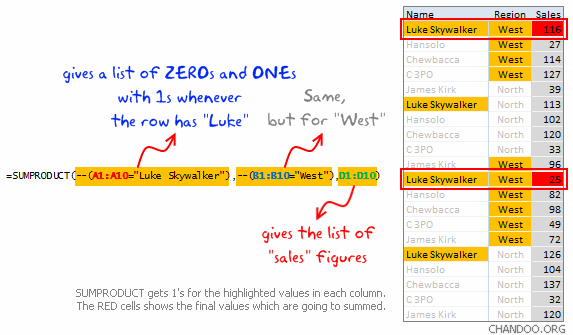I learned about SUMPRODUCT and Double Unary Operators recently. Any test used in array formulas returns an array of TRUE/FALSE values and in order to use the results of that test in a calculation an operator is required to convert those TRUE/FALSE values to 1/0’s. SUMPRODUCT is a built-in Excel function which manages to perform the operation without the use of an array formula. Traditionally I would use an array formula to figure out the total sales of Luke Skywalker in the West region. The formula would look like this:

``````{= SUM((A1:A10="Luke Skywalker") * (B1:B10="West) * (C1:C10))}
``````

Note that I have assumed the sales figures are actually in column C (the image is assuming column D).Excel is taking three arrays and multiplying them together while simultaneously performing two tests to establish the first two arrays. Mathematically we have `{1, 0, 0, 0, 0, 1, 0, 0, 0, 0, 1, 0, 0, 0, 0, 1, 0, 0, 0, 0}` for the Luke Skywalker test.

The reason SUMPRODUCT is the better choice than an array formula is it is a built in function, it does not rely on the user recognizing an array formula (Ctrl-Shift-Enter on the original formula I would have used), and the general format of the function makes it easy to figure out what is going on.

The trick here is the addition of the double unary operators on the two tests. I had forgotten about unary operators - you can read up on this elsewhere but bottom line is that the double unary operators cause Excel to convert True/False values into 1’s / 0’s which can be multiplied by values in SUMPRODUCT. So the equivalent SUMPRODUCT formula for this situation would look like:

``````= SUMPRODUCT(--(A1:A10="Luke Skywalker"), --(B1:B10="West), C1:C10)
``````

Note that those are indeed double dashes in the SUMPRODUCT formula above, the double unary operators that make the formula work.

http://www.xldynamic.com/source/xld.SUMPRODUCT.html

http://chandoo.org/wp/2009/11/10/excel-sumproduct-formula/

http://www.excelforum.com/excel-general/731580-double-dash-what-is-it.html

http://static.chandoo.org/img/n/sumproduct-tutorial-and-help.png